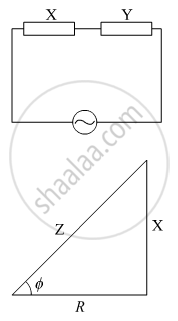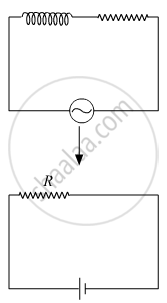Advertisement Remove all ads

# An ac circuit consists of a series combination of circuit elements X and Y. The current is ahead of the voltage in phase by pi/4 - Physics

Advertisement Remove all ads
Advertisement Remove all ads
Advertisement Remove all ads
Numerical

An ac circuit consists of a series combination of circuit elements X and Y. The current is ahead of the voltage in phase by pi/4. If element X is a pure resistor of 100 Ω,

(a) name the circuit element Y.

(b) calculate the rms value of current, if rms of voltage is 141 V.

(c) what will happen if the ac source is replaced by a dc source

Advertisement Remove all ads

#### Solution

(a) We have,Φ = π/4

tan Φ = 1

tanphi  = "Reactance"/"Resistance"

Reactance = Resistance

Y = 100 Ω

As, ϕ > 0°, means voltage ahead of current Hence element Y is an Inductor.

(b)

"R.m.s value of current" = "R.m.s value of voltage"/"Impedance"

"i"_"Rms" = 141/sqrt((100)^2+ (100)^2)

"i"_"Rms" = 141/(100sqrt2) = 141/(100 xx 1.41)

iRms  = 1 A

(c)  If the Ac is Replace by DC, then the Inductor will behave as a short circuit, and the circuit will be purely Resistive.Concept: Combination of Capacitors
Is there an error in this question or solution?

#### Video TutorialsVIEW ALL 

Advertisement Remove all ads
Share
Notifications

View all notifications

Forgot password?